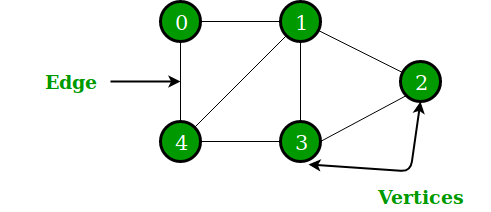# Applications of Graph Data Structure

• Difficulty Level : Easy
• Last Updated : 13 Jun, 2022

A graph is a non-linear data structure, which consists of vertices(or nodes) connected by edges(or arcs) where edges may be directed or undirected.• In Computer science graphs are used to represent the flow of computation.
• Google maps uses graphs for building transportation systems, where intersection of two(or more) roads are considered to be a vertex and the road connecting two vertices is considered to be an edge, thus their navigation system is based on the algorithm to calculate the shortest path between two vertices.
• In Facebook, users are considered to be the vertices and if they are friends then there is an edge running between them. Facebook’s Friend suggestion algorithm uses graph theory. Facebook is an example of undirected graph.
• In World Wide Web, web pages are considered to be the vertices. There is an edge from a page u to other page v if there is a link of page v on page u. This is an example of Directed graph. It was the basic idea behind Google Page Ranking Algorithm.
• In Operating System, we come across the Resource Allocation Graph where each process and resources are considered to be vertices. Edges are drawn from resources to the allocated process, or from requesting process to the requested resource. If this leads to any formation of a cycle then a deadlock will occur.
• In mapping system we use graph. It is useful to find out which is an excellent place from the location as well as your nearby location. In GPS we also use graphs.
• Facebook uses graphs. Using graphs suggests mutual friends. it shows a list of the f following pages, friends, and contact list.
• Microsoft Excel uses DAG means Directed Acyclic Graphs.
• In the Dijkstra algorithm, we use a graph. we find the smallest path between two or many nodes.
• On social media sites, we use graphs to track the data of the users. liked showing preferred post suggestions, recommendations, etc.

Thus the development of algorithms to handle graphs is of major interest in the field of computer science.

My Personal Notes arrow_drop_up